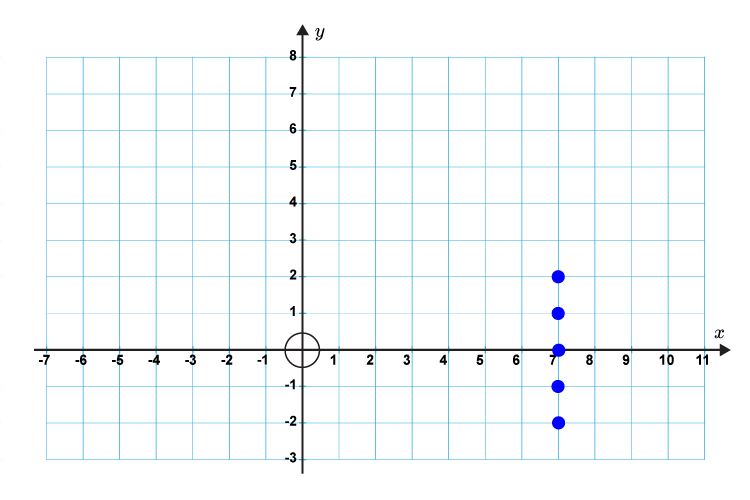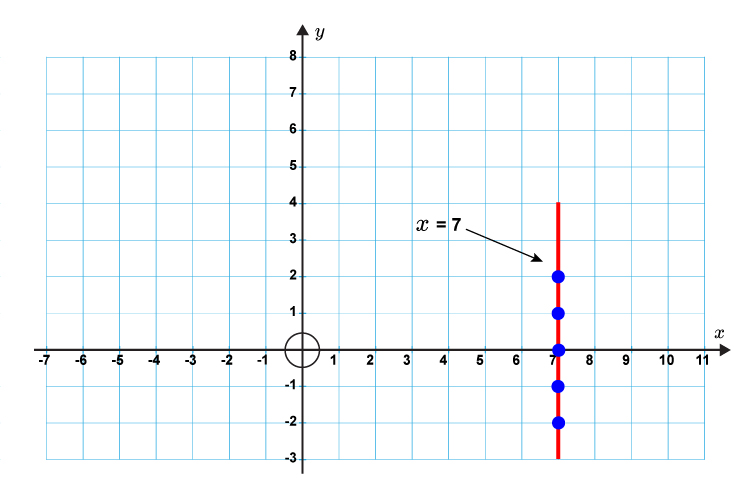# Equation of a vertical line

The equation of a vertical line is:

x=  The same number

Example

x = 7

No matter what value y is, x is 7.

y = 2              x = 7

y = 1              x = 7

y = 0              x = 7

y = -1        x = 7

y = -2        x = 7

The graph would be plotted as follows.And would look like this.This is a vertical line.